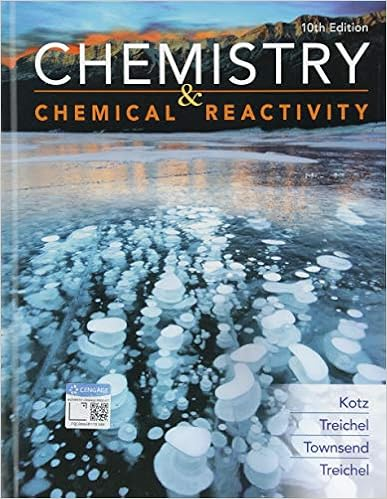# APchp5supprob - AP Chemistry Chapter 5 Solution Chemistry...

• Notes
• UltraLightningCobra8457
• 1

This preview shows page 1 out of 1 page.

##### We have textbook solutions for you!
The document you are viewing contains questions related to this textbook.The document you are viewing contains questions related to this textbook.
Chapter 1 / Exercise 49
Chemistry & Chemical Reactivity
Kotz/TreichelExpert Verified
AP Chemistry Chapter 5 – Solution Chemistry Additional Sample Problems 1. Identify the oxidizing agent and the reducing agent in the following reaction: 4Au(s)+ 8CN-(aq)+ O2(g)+ 2H2O(l)→ 4[Au(CN)2]-(aq)+ 4OH-(aq)2. A 0.235g sample of a solid that is 92.5% NaOH and 7.5% Ca(OH)2, by mass, requires 45.6mL of a HCl(aq)solution for its titration. What is the molarity of the HCl(aq)3. what molarity of NaF(aq)corresponds to a fluoride ion content of 0.9mg F-/L, the federal government’s recommended limit for fluoride ion in drinking water? 4. Predict whether a reaction is likely to occur in each of the following cases. If so, write a net ionic equation. a) HI(aq)+ Zn(NO3?
##### We have textbook solutions for you!
The document you are viewing contains questions related to this textbook.The document you are viewing contains questions related to this textbook.
Chapter 1 / Exercise 49
Chemistry & Chemical Reactivity
Kotz/TreichelExpert Verified
•••